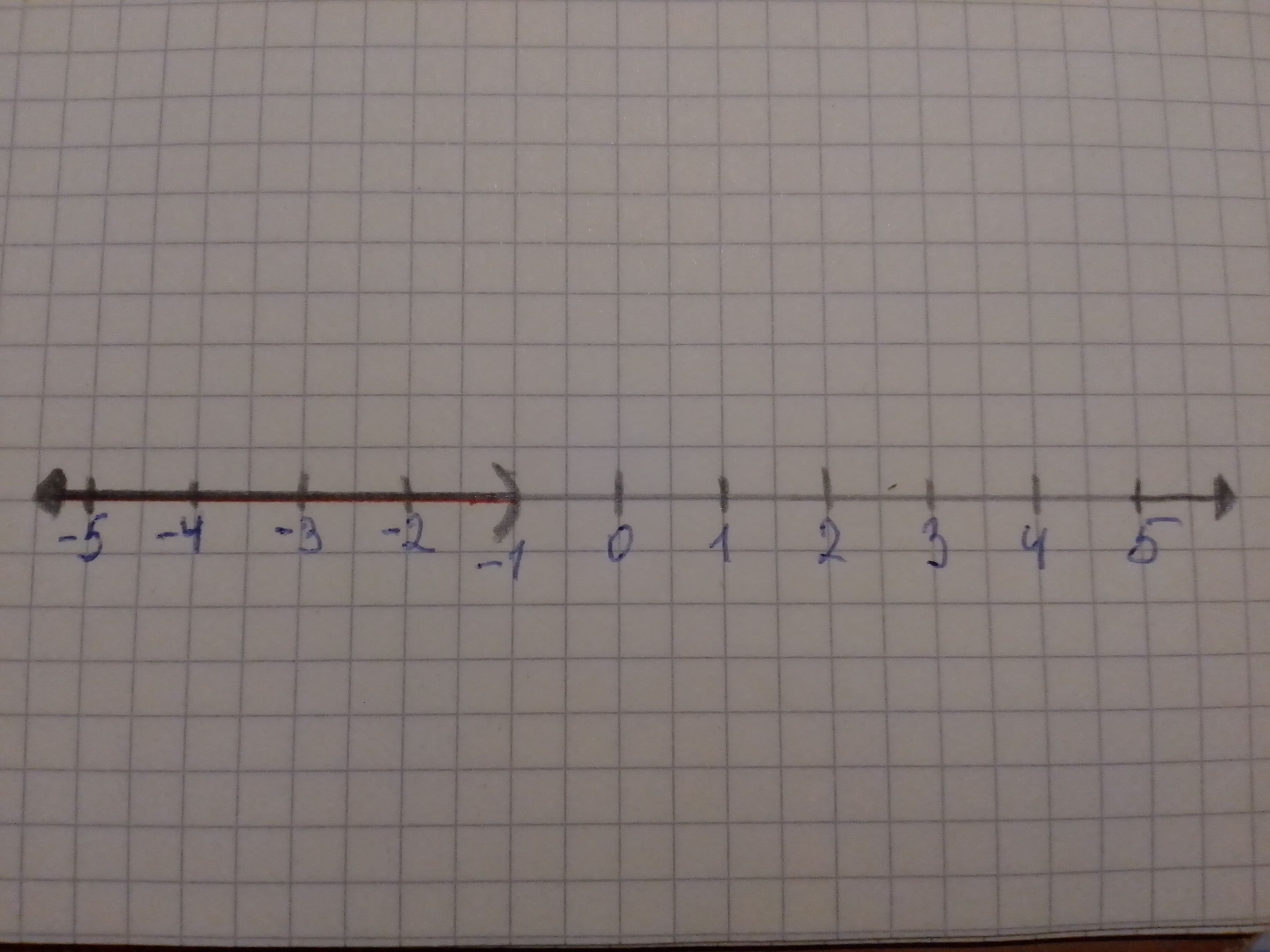# The of the graph of the inequality and the interval notation of the solution. The given inequality equation is, displaystyle-{1}>{x}Tobias Ali 2021-01-08 Answered
The of the graph of the inequality and the interval notation of the solution.
The given inequality equation is,
$-1>x$
You can still ask an expert for help

• Questions are typically answered in as fast as 30 minutes

Solve your problem for the price of one coffee

• Math expert for every subject
• Pay only if we can solve itBenedict

Concept used:
Consider the following steps to solve the linear equation in one variable:
If an equation contains fractions or decimals, multiply both sides by the LCD to clear the equation of fractions or decimals.
Use the distributive property to remove parentheses if they are present.
Simplify each side of the equation by combining like terms.
Get all variable terms on one side and all numbers on the other side by using the addition property of inequality.
Get only the variable using the inequality multiplication property.
The given inequality equation is,
$-1>x$
$-1-x>x-x$
$-1-x+1>0+1$
Simplify further,
$-x>1$
$\frac{-x}{-1}<\frac{1}{-1}$
$x<-1$
Consider the following graph of inequality,Figure (1)
The interval notation of the inequality is written as $\left(-\mathrm{\infty },-1\right)$
Hence, the graph of the inequality is shown in figure (1) and the interval notation is $\left(-\mathrm{\infty },-1\right)$